# Obeleži sve kategorije koje odgovaraju problemu

### Još detalja - opišite nam problem

Uspešno ste prijavili problem!
Status problema i sve dodatne informacije možete pratiti klikom na link.
Nažalost nismo trenutno u mogućnosti da obradimo vaš zahtev.
Molimo vas da pokušate kasnije.

# Working with Blocks from the Math and Variables Categories¶

MakeCode supports four basic arithmetic operations: - addition ( + ), - subtraction ( - ), - multiplication ( * ) and - division ( / ).

The blocks that enable computation are called arithmetic operators. They are located in the category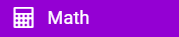.

Arithmetic operators return a NUMBER (the result of an arithmetic operation).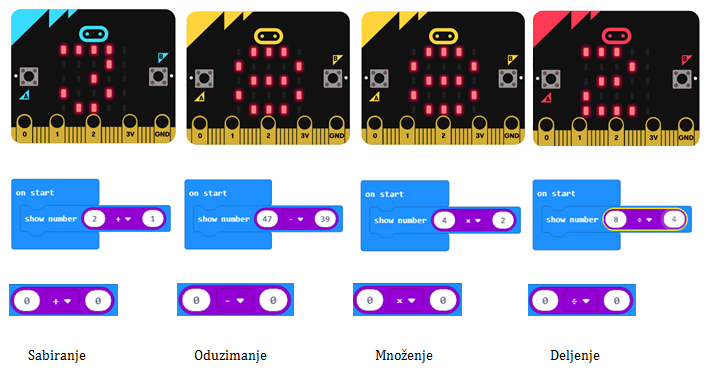You can use the result (number) returned by an arithmetic operator as an input value for blocks, which accept numbers. This can be clearly seen in the figure above. The show number … block accepts a number as an input and displays it on the screen.

We put before you a more complex arithmetic expression: ( 2 + 1 ) * ( 12 - 10 ). In MakeCode, calculating the result might look like this: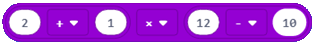By analyzing the complex expression, we can conclude that it consists of smaller units - interim results.

The sum of (2 + 1) is one interim result. The difference of (12-10) is the second interim result. We will get the result of the whole expression when we multiply the sum and the difference (sum * difference).

In programming, it is convenient to use the interim results (interim values), which we call variables. You can think of variables as spaces in computer memory, similar to boxes, in which the interim results are stored. Variables have names.

When you want to use the value of a specific variable in a program, it is enough just to indicate its name.

In MakeCode, you create variables in the category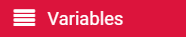.

We create variables by clicking on the Make a variable button (2) in the Variable category (1), entering the name of the variable (3), in our case the name Counter, and then click on the OK button (4).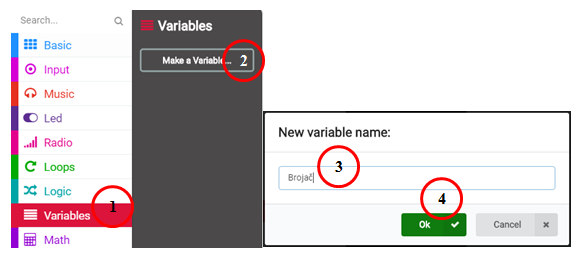Clearly, you can present our (2 + 1) * (12 - 10) expression differently. By creating two variables: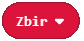and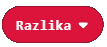.

We will set the initial value of the Counter variable to be 0. This can be done by dragging the block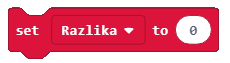from the categoryinto the block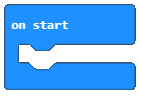.

The final look of the code: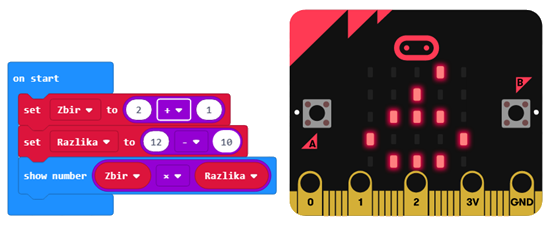Q-11: Create a program, which has two variables: x and y. The set value of the variable x should be 2 (x = 2), and the set value of the variable y should be 4 (y = 4). When the program runs the block shown in the figure below, the result will be: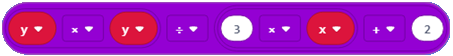• 2.
• 10.
• The answer is not correct!
• 4.
• The answer is not correct!

Let’s use arithmetic operators and variables to create a program, which calculates the perimeter and area of ​​a square.

To calculate the area of ​​a square, it is necessary to define the length of the side a, whose value will change randomly, each time the user presses button A, and when button B is pressed, the program will calculate the perimeter and the area of the given square.

At the very beginning, we need to create the variables a, P and A, which represent the side, the perimeter and the area of the square, respectively.

The random change of the value of the variable will be defined by the use of the block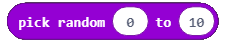.

The look of the code when the initial value is defined and set in the block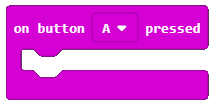is shown below: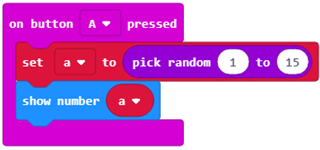To see the value of the length of the side a, we can drag the display block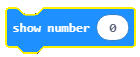.

The look of the block: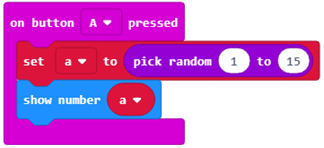To calculate the perimeter and the area of ​​a square, we must remind ourselves that the perimeter of a square is 4*the length of the side (4*a) and that the area of a square is the product of the length of its sides (a*a). We use multiplication for calculating this. The look of the block for calculating the perimeter and the area of a square is: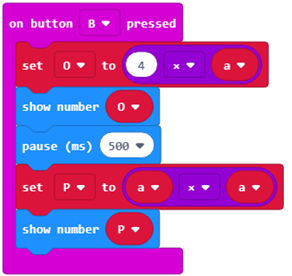To test the program, we will run it in the simulator by clicking the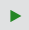button.

Q-12: Study the block carefully: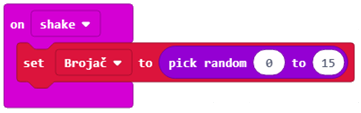What will be the value of the Counter variable when a movement is made (shake)?

• Any value from the interval 0 to 15, not including 0.
• The answer is not correct!
• Any value from the interval 0 to 15, including those values.